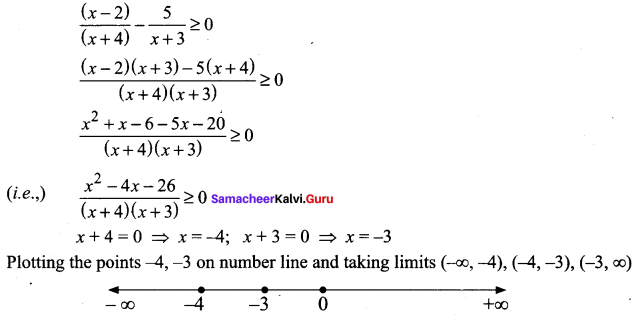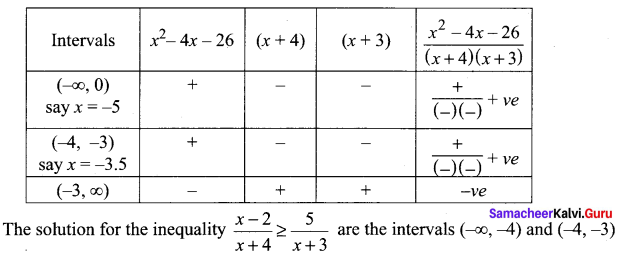# Samacheer Kalvi 11th Maths Solutions Chapter 2 Basic Algebra Ex 2.8

## Tamilnadu Samacheer Kalvi 11th Maths Solutions Chapter 2 Basic Algebra Ex 2.8

Question 1.
Find all values of x for which $$\frac{x^{3}(x-1)}{(x-2)}$$ > 0
Solution: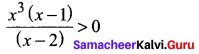Now we have to find the signs of
x3, x – 1 and x – 2 as follows
x3 = 0; x – 1 = 0 ⇒ x = 1; x – 2 = 0 ⇒ x = 2
Plotting the points in a number line and finding intervals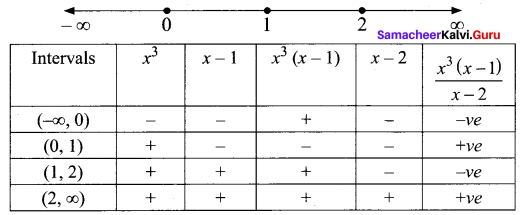So the solution set = (0, 1) ∪ (2, ∞)Question 2.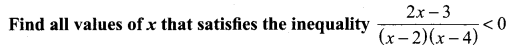Solution: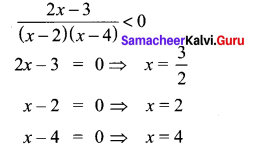Plotting the points 3/2, 2 and 4 on the number line and taking the intervals.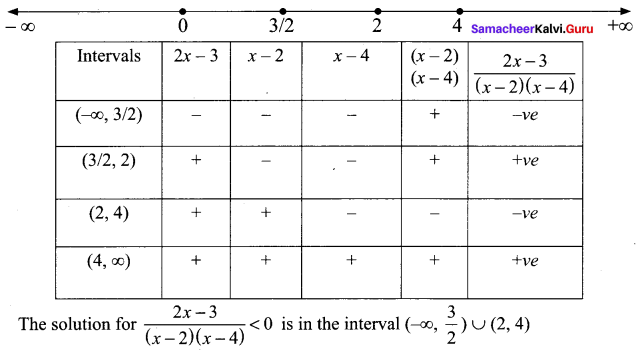Question 3.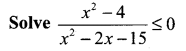Solution: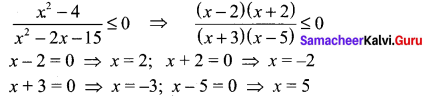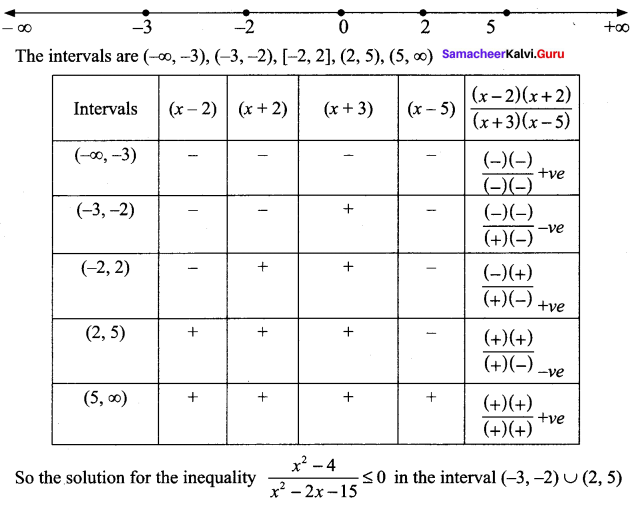### Samacheer Kalvi 11th Maths Solutions Chapter 2 Basic Algebra Ex 2.8 Additional Questions

Question 1.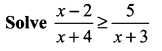Solution: